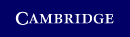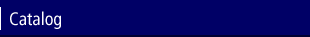Home > Catalog > Understanding ProbabilitySearch this bookDetails

• 42 b/w illus. 22 tables 400 exercises
• Page extent: 452 pages
• Size: 228 x 152 mm
• Weight: 0.608 kg

Library of Congress

• Dewey number: 519.2
• Dewey version: 22
• LC Classification: QA273 .T48 2007
• Probabilities
• Mathematical analysis
• Chance
• Waarschijnlijkheidstheorie.--gtt

Library of Congress Record

Paperback

(ISBN-13: 9780521701723)

Replaced by 9781107658561

\$48.00

In this fully revised second edition of Understanding Probability, the reader can learn about the world of probability in an informal way. The author demystifies the law of large numbers, betting systems, random walks, the bootstrap, rare events, the central limit theorem, the Bayesian approach and more. This second edition has wider coverage, more explanations and examples and exercises, and a new chapter introducing Markov chains, making it a great choice for a first probability course. But its easy-going style makes it just as valuable if you want to learn about the subject on your own, and high school algebra is really all the mathematical background you need.

Contents

Preface; Introduction; Part I. Probability in Action: 1. Probability questions; 2. The law of large numbers and simulation; 3. Probabilities in everyday life; 4. Rare events and lotteries; 5. Probability and statistics; 6. Chance trees and Bayes' rule; Part II. Essentials of Probability: 7. Foundations of probability theory; 8. Conditional probability and Bayes; 9. Basic rules for discrete random variables; 10. Continuous random variables; 11. Jointly distributed random variables; 12. Multivariate normal distribution; 13. Conditional distributions; 14. Generating functions; 15. Markov chains; Appendix; Recommended readings; Answers to odd-numbered problems; Bibliography.

Review

"An excellent book for the study of basic probability events... This book is an excellent choice for advanced courses in probability for math majors who have completed the calculus sequence."
Charles Ashbacher, Journal of Recreational Mathematics